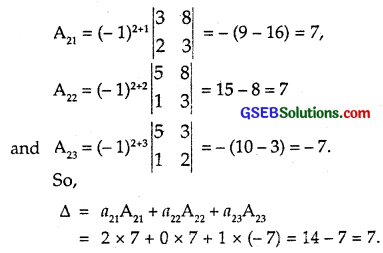# GSEB Solutions Class 12 Maths Chapter 4 Determinants Ex 4.4

Gujarat Board GSEB Textbook Solutions Class 12 Maths Chapter 4 Determinants Ex 4.4 Textbook Questions and Answers.

## Gujarat Board Textbook Solutions Class 12 Maths Chapter 4 Determinants Ex 4.4Write minors and cofactors of elements of the following determinants:

Question 1.
(i) $$\left|\begin{array}{cc} 2 & -4 \\ 0 & 3 \end{array}\right|$$
(ii) $$\left|\begin{array}{cc} a & c \\ b & d \end{array}\right|$$
Solution:
(i) ∆ = $$\left|\begin{array}{cc} 2 & -4 \\ 0 & 3 \end{array}\right|$$
Minor of a11 is M11 = 3,
Minor of a12 is M12 = 0,
Minor of a21 is M21 = – 4,
Minor of a22 is M22 = 2.
Cofactor of a11 = A11 = (-1)1+1 M11 = (-1)² x 3 = 3,
Cofactor of a12 = A21 = (-1)2+1M12 = 0,
Cofactor of a21 = A21 = (-1)2+1 M21 = – (- 4) = 4,
Cofactor of a22 = A22 = (-1)2+2 M22 = 2.

(ii)Question 2.
(i) $$\left|\begin{array}{lll} 1 & 0 & 0 \\ 0 & 1 & 0 \\ 0 & 0 & 1 \end{array}\right|$$
(ii) $$\left|\begin{array}{ccc} 1 & 0 & 4 \\ 3 & 5 & -1 \\ \theta & 1 & 2 \end{array}\right|$$
Solution:Question 3.
Using cofactors of elements of second row, evaluate
∆ = $$\left|\begin{array}{rrr} 5 & 3 & 8 \\ 2 & 0 & 1 \\ 1 & 2 & -3 \end{array}\right|$$.
Solution:Question 4.
Using cofactors of elements of third column, evaluate
∆ = $$\left|\begin{array}{rrr} 1 & x & yz \\ 1 & y & zx \\ 1 & z & xy \end{array}\right|$$.
Solution:
Elements of third column are yz, zx and xy.
A13 = (-1)1+3$$\left|\begin{array}{ll} 1 & y \\ 1 & z \end{array}\right|$$ = z – y
A23 = (-1)2+3$$\left|\begin{array}{ll} 1 & x \\ 1 & z \end{array}\right|$$ = – (z – x) = – z + x
A33 = (-1)3+3$$\left|\begin{array}{ll} 1 & x \\ 1 & y \end{array}\right|$$ = (y – x)
∴ ∆ = a13A13 + a23A23 + a33A33
= yz(z – y) + zx(- z + x) + xy(y – x)
= yz² – y²z – xz² + x²z + xy² – x²y
= (- y²z + yz²) + (xy² – xz²) + (- x²y + x²z)
= – yz(y – z) + x(y² – z²) – x²(y – z)
= (y-z)[- yz + (y + z) – x²]
= (y-z)[-yz + xy+ xz-x²]
= (y – z)[xy – yz – x² + xy]
= (y – z)[z(x – y) – x(x – y)]
= (y – z)(x – y)(z – x)
= (x – y)(y – z)(z – x).Question 5.
If A = $$\left|\begin{array}{lll} a_{11} & a_{12} & a_{13} \\ a_{21} & a_{22} & a_{23} \\ a_{31} & a_{32} & a_{33} \end{array}\right|$$ and Aij is the cofactor of aij, then value of ∆ is given by
(A) a11A31 + a12A32 + a13A33
(B) a11A11 + a12A21 + a13A31
(C) a21A11 + a22A12 + a23A13
(D) a11A11 + a21A21 + a31A31
Solution:
A = Sum of the products of elements of a row or column with the corresponding cofactors.
Here, Part (D) is the required answer, because elements of 1 column are multiplied by their corresponding cofactors.
So, a11A11 + a21A21 + a31A31.